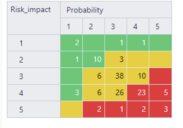# Merge two column in one

Hi,
I am trying to create a heat map view, initially was successful as below image:However when more projects are adding in, they have a different data as you can see below. is there a way that I can create a calculated member to merge “1” and “1-extremly unlikely (<10%)” into column or row as “1” only?

I tried with

CASE [Risk_impact].[All Risk_impacts].Value
When “1-extremly unlikely (<10%)” THEN 1
END
but got error below:
Formula is not valid:
No function matches signature ‘CASE WHEN THEN … END’

Any suggestions?

thanks!

You might want to clean up the data. The best way to do this is JavaScript code in custom field advanced settings. The idea for the code would be to check the risk value and get from it only the numeric part like 1, 2, 3, 4, and 5.

The JavaScript calculated custom field that merges similar values keeping only the numeric part might look like this:

``````[jira.customfield_risklevel]
name = "Risk Level (merged)"
data_type = "string"
dimension = true
javascript_code = '''
if(issue.fields.customfield_NNNNN ) {
var risklevel = issue.fields.customfield_NNNNN.match(/\d+/);
issue.fields.customfield_risklevel = risklevel;
}
'''
``````

Before using this code, please test it on an individual issue and if the custom field value is accessible directly (as in the example) or it is one level deeper in the JSON object (`issue.fields.customfield_NNNNN.value`). More details on JavaScript calculated custom fields and how to validate them are described in the documentation: JavaScript calculated custom fields.

If JavaScript calculated custom field is not an option for you, an alternative is calculated member for each risk level you would like to merge. For merging values, use function Aggregate() and list similar values. See the documentation on Calculated members in other dimensions.

For example, define a new calculated member in Risk_impact dimension to group values “2” and “2 - Minor scope reduction &/or Minor impact”:

``````Aggregate({
[Risk_impact].,
[Risk_impact].[2 - Minor scope reduction &/or Minor impact]
})
``````

Best,
Zane / support@eazyBI.com